## Spirograph

A Hypotrochoid generated by a fixed point on a Circle rolling inside a fixed Circle. It has parametric equations,(1)(2)

whereis the radius of the fixed circle,is the radius of the rotating circle, andis the offset of the edge of the rotating circle. The figure closes only if,, andare Rational. The equations can also be written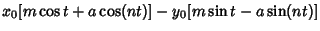(3)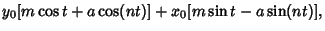(4)

where the outer wheel has radius 1, the inner wheel a radius, the pen is placedunits from the center, the beginning is atradians above the-axis, and(5)(6)(7)(8)

The following curves are for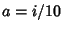, with, 2, ..., 10, and.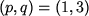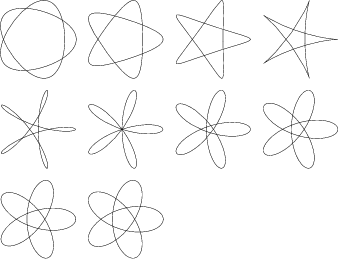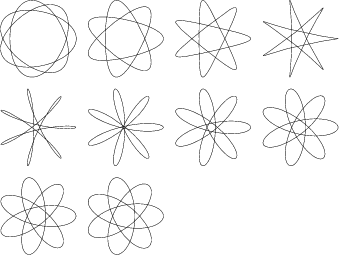Additional attractive designs such as the following can also be made by superposing individual spirographs.